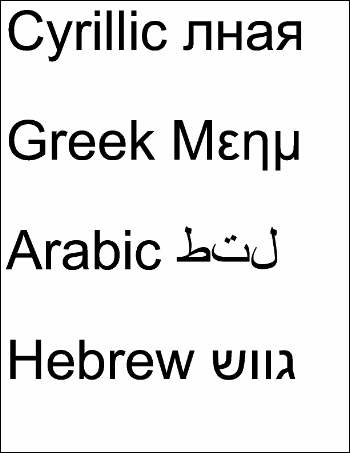eForm FDF ExampleThis example shows how to extract Unicode annotation values from an eForm FDF file.Src

 First, we create an ABCpdf Doc object and read in our FDF file. ```Set theFDF = Server.CreateObject("ABCpdf12.Doc") theFDF.Read "c:\mypdfs\form.fdf"```Dest

 We find out how many items there are in the FDF file and prepare to iterate through them. ```theValues = "" theLastID = CInt(theFDF.GetInfo(0, "Count"))```We go through each item. We check to see whether it is an annotation. If it is, we check to see if the annotation type is text. If we have found a text annotation, we extract the content and add the value to our list. ```For i = 0 To theLastID   theType = theFDF.GetInfo(i, "Type")   If theType = "anno" Then     If theFDF.GetInfo(i, "SubType") = "Text" Then       theCont = theFDF.GetInfo(i, "Contents")       theValues = theValues & theCont & vbCrLf & vbCrLf     End If   End If Next```Finally, we add the Unicode text to a new document and save it. ```Set theDoc = Server.CreateObject("ABCpdf12.Doc") theDoc.Font = theDoc.EmbedFont("Arial", "Unicode", False, True) theDoc.FontSize = 96 theDoc.Rect.Inset 10, 10 theDoc.AddText theValues theDoc.Save "c:\mypdfs\fdf.pdf"```This is the kind of PDF you might expect to produce.fdf.pdf